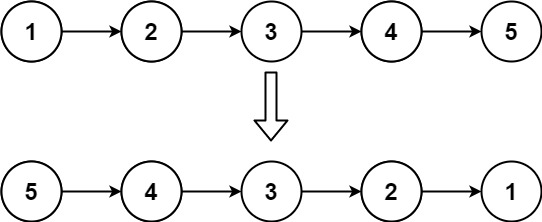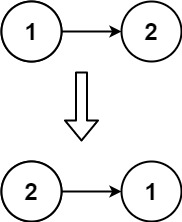# GeetCode Hub

Given the `head` of a singly linked list, reverse the list, and return the reversed list.

Example 1:```Input: head = [1,2,3,4,5]
Output: [5,4,3,2,1]
```

Example 2:```Input: head = [1,2]
Output: [2,1]
```

Example 3:

```Input: head = []
Output: []
```

Constraints:

• The number of nodes in the list is the range `[0, 5000]`.
• `-5000 <= Node.val <= 5000`

Follow up: A linked list can be reversed either iteratively or recursively. Could you implement both?

/** * Definition for singly-linked list. * public class ListNode { * int val; * ListNode next; * ListNode() {} * ListNode(int val) { this.val = val; } * ListNode(int val, ListNode next) { this.val = val; this.next = next; } * } */ class Solution { public ListNode reverseList(ListNode head) { } }